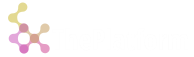# Signals / Error handling

Signalling in O means throwing an error. The idea is similar to raising exceptions in other languages. That is signals are meant to break normal calculation flow and report an error.

Trapping a signal means running a function and expecting any error occurance. Conceptually it is similar to "catch" clause in other languages.

## Signals

Technically signalling is done using monadic verb `'`. Its argument is any value describing error.

``````o)a:10; { { '(`s;1); set[`a;0] }[] }[]
'`s
1
o)a
10
o)
``````

## Trap

Trapping is done using `@` and `.` triads.

The `@` triad applies its first argument (a monadic function) to its second argument. When function fails or signals, the entire expression becomes equal to third argument.

``````o)@[{x};1;`err2]
1
o)@[{'x};`err1;`err2]
`err2
o)
``````

Another possible usage is trapping with a function as the third argument. When signal occurs, it leads to execution of the third argument function with signal value as an argument.

``````o)@[{'x};`err1;{x}]
`err1
o)
``````

Runtime errors are also caught using traps. Trapping runtime errors results in a signal value with the error text message as its value.

``````o)@[{x+y};1;{x}]
"** exec error: +: invalid args [1;]
|--> [REPL::1] @[{x+y};1;{x}]
^"
o)
``````

The `.` triad is pretty much the same expression except its second argument can be a list/vector. It is used as several arguments for a trapped function. Thus, it allows using a function with arity greater than 1.

``````o).[+;(1;2);`err2]
3
o).[{'y};(1;`err1);{x}]
`err1
o)
``````